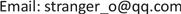1. 引言

2. 总体方案

3. 图像预处理部分

4. 定位算法

1) 首先，选定模板，它必须能区分每个阵列单元，拥有的特征需要与单元内有较高相关性而与单元外(单元间隙)有较低的相关性。

2) 计算模板与待匹配子图的相关性或差异性，通过在某一位置的邻域内寻找相关性最大或差异性最小的子图。

3) 遍历搜索整张图片后得到所有子图最佳的定位点。

D ( i , j ) = ∑ m = 1 M ∑ N = 1 N [ S i j ( m , n ) − T ( m , n ) ] 2

c c ( i , j ) = ∑ m = 1 M ∑ n = 1 N S i j ( m , n ) × T ( m , n ) ∑ m = 1 M ∑ N = 1 N [ S i j ( m , n ) ] 2 ∑ m = 1 M ∑ N = 1 N [ T ( m , n ) ] 2

5. 结论